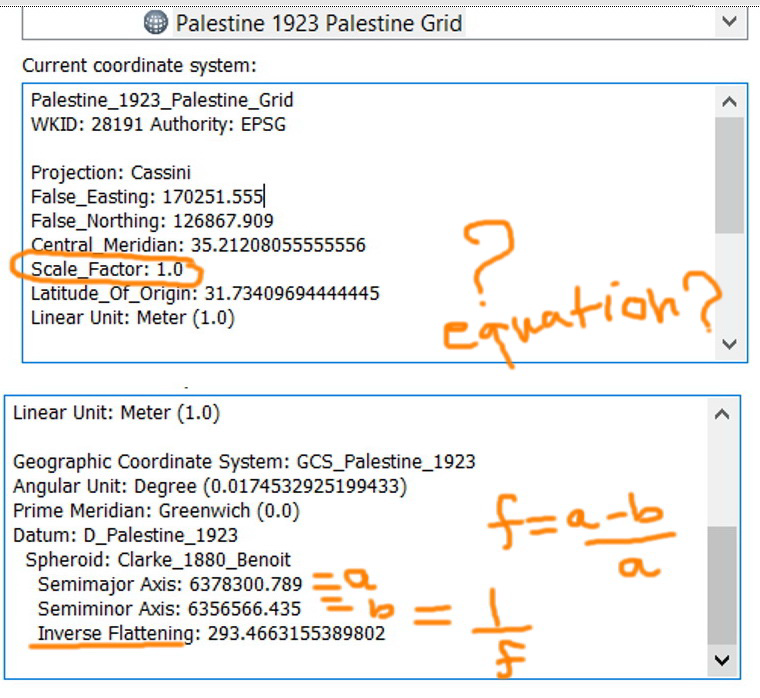# How to calculate\determine the “Scale Factor” of a projection coordinate system?

4076
2
10-23-2017 02:12 PMEsteemed Contributor

How to calculate\determine the “Scale Factor” of a projection coordinate system?

I couldn’t figure out if there are equations based on which the “Scale Factor” is calculated the same way we do for the flatness ((a-b)/a)----------------------------------------
Jamal Numan
Geomolg Geoportal for Spatial Information
Ramallah, West Bank, Palestine
2 RepliesbyEsri Notable Contributor

I'm sorry for the delay in responding.

When you see a scale factor listed among the projection parameters, it's a defining characteristic of the projected coordinate system. It is not the scale/distortion-at-a-point that can be calculated via a Tissot Indicatrix or other calculation. At some point, we do plan to add those types of calculations, but don't have them yet.

So for instance, in a Cassini projection, the scale factor sets the scale along the central meridian.

In a UTM zone, where the scale factor is 0.9996, that's the scale along the central meridian which means there are two lines (they are not quite longitude lines) approximately 180 km west and east of the central meridian that have scale = 1.

MelitaEsteemed Contributor

Thank you Melita for the input. This is very useful.

----------------------------------------
Jamal Numan
Geomolg Geoportal for Spatial Information
Ramallah, West Bank, Palestine